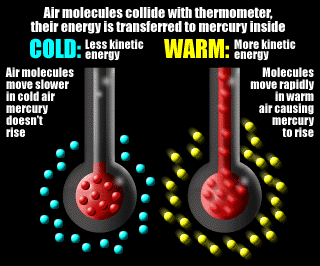## miércoles, 11 de noviembre de 2009

### Temperatures scales

Temperature is a measure of molecular motion

Air temperature is one of those things that everyone is familiar with, which turns out to be more complicated than it might seem at first.

A thermometer actually measures the average kinetic energy of the various gas molecules that make up the air around it - let's call them "air molecules."As you can see in the graphic on the left, air molecules in colder air move slowly compared to those in warmer air. The kinetic energy of an air molecule is directly proportional to the velocity of the molecule.

This means that colder air has less kinetic energy than warmer air.
When air molecules collide with a thermometer, kinetic energy is transferred from the air molecules to the glass and then to the mercury molecules inside the thermometer.
As the mercury molecules begin moving faster they move farther apart, pushing the mercury up in the thermometer.

In colder air, the energy from the air molecules colliding with the thermometer transferring to the mercury molecules is less than the energy from warmer air. As a result, the mercury molecules move slower in the colder air and the mercury inside the thermometer does not expand as far up the tube as it does in the warmer air.

Temperature is the level of heat in a gas, liquid, or solid. Three scales are commonly used for measuring temperature. The Celsius and Fahrenheit scales are the most common. The Kelvin scale is primarily used in scientific experiments.

Celsius Scale
The Celsius scale was invented in 1742 by the Swedish astronomer, Anders Celsius. This scale divides the range of temperature between the freezing and boiling temperatures of water into 100 equal parts. You will sometimes find this scale identified as the centigrade scale. Temperatures on the Celsius scale are known as degree Celsius (ºC).

Fahrenheit Scale
The Fahrenheit scale was established by the German-Dutch physicist, Gabriel Daniel Fahrenheit, in 1724. While many countries now use the Celsius scale, the Fahrenheit scale is widely used in the United States. It divides the difference between the melting and boiling points of water into 180 equal intervals. Temperatures on the Fahrenheit scale are known as degree Fahrenheit (ºF).

Kelvin Scale
The Kelvin scale is named after William Thompson Kelvin, a British physicist who devised it in 1848. It extends the Celsius scale down to absolute zero, a hypothetical temperature characterized by a complete absence of heat energy. Temperatures on this scale are called Kelvins (K).

Converting Temperatures
It is sometimes necessary to convert temperature from one scale to another. Here is how to do this.

To convert from ºC to ºF use the formula: ºF = ºC x 1.8 + 32.
To convert from ºF to ºC use the formula: ºC = (ºF-32) / 1.8.
To convert from K to ºC use the formula: ºC = K – 273.15
To convert from ºC to K use the formula: K = ºC + 273.15.
To convert from ºF to K use the formula: K = 5/9 (ºF – 32) + 273.15.
To convert from K to ºF use the formula: ºF = 1.8(K – 273.15) + 32.

#### Publicar un comentario

Hola, gracias por dejar tu comentario.